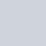This Math Problem Was Most Searched by Indians on Google in 2021 – Do You Know the Answer?Published

on

This Math Problem Was Most Searched by Indians on Google in 2021 – Do You Know the Answer?

This Math Problem Was Most Searched by Indians on Google in 2021 – Do You Know the Answer?
What is the factorial of hundred’ was one of the top queries by Indians on Google in 2021. Image for representation. Credits: Pexels via Canva.
‘What is the factorial of hundred’ was one of the top queries by Indians on Google in 2021. Image for representation. Credits: Pexels via Canva.
‘What is the factorial of hundred’ was one of the top queries by Indians on Google in 2021.

Math only has two sides: You either love it or hate it(but you can’t live without it.) From as basic calculation as return change from a shopkeeper to calculating the interest in your bank balances, when schoolteachers said ‘You’ll need Math every day of your life’ they really meant it. We may be passing days without using Tan Q, but we’re definitely thankful to know the basics. But what about math that’s not as easy? Indians turn out, have had to Google the answers to some equations to math, because it was perhaps not that easy to figure out on our own, or perhaps to double-check whether the method on paper is actually the correct answer backed up by Google.

In Google’s Year in Review 2021 for India, one single math problem was high on the list of things Indians searched for in the year.

‘What is the factorial of hundred’ was #2 on India’s top searches in 2021. The search term peaked between May 30 to June 5, 2021 when questions about the answer shot up.

So, what’s the answer to ‘What is the factorial of a hundred?’. To ELI5 it, we first need to understand what a factorial is.

What is a factorial? According to the Britannica definition “factorial, in mathematics, the product of all positive integers less than or equal to a given positive integer and denoted by that integer and an exclamation point.” To put it into numbers, if we were trying to find the factorial for 5, it would be written as 5!
What would the answer be? 5! = 1x2x3x4x5 or 120.

Similar, for 6! it would be 1x2x3x4x5x6 or 720.

So what is the factorial for 100? We can calculate it by 1x2x3x4x5x6x7… all the way to 100. The long answer is:

93326215443944152681699238856266700490715968264381621468592963895217599993229915608941463976156518286253697920827223758251185210916864000000000000000000000000.
The short answer, and the easier to define answer, is 9.3326215443944E+157 (approximately.)